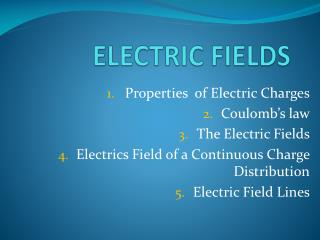DownloadDownload PresentationELECTRIC FIELDS

# ELECTRIC FIELDS

Download Presentation## ELECTRIC FIELDS

- - - - - - - - - - - - - - - - - - - - - - - - - - - E N D - - - - - - - - - - - - - - - - - - - - - - - - - - -
##### Presentation Transcript

1. ELECTRIC FIELDS Properties of Electric Charges Coulomb’s law The Electric Fields Electrics Field of a Continuous Charge Distribution Electric Field Lines

2. Why each individual hair stand-up?? Each individual hair on their heads becomes charged and exerts a repulsive force on the other hairs, resulting in the “stand-up’’ hairdos that you see here

3. 1. Properties of Electric Charges A negatively charged rubber rod suspended by a thread is attracted to a positively charged glass rod. A negatively charged rubber rod is repelled by another negatively charged rubber rod.

4. When a glass rod is rubbed with silk, electrons are transferred from the glass to the silk. Because of conservation of charge, each electron adds negative charge to the silk, and an equal positive charge is left behind on the rod. Also, because the charges are transferred in discrete bundles, the charges on the two objects are \$e, or \$2e, or \$3e, and so on.

5. We conclude that electric charge has the following important properties: • There are two kinds of charge in nature; charges of the same sign repel one another and charges of opposite sign attract one another • Total charge in an isolated system is conserved • Charges is quantized

6. A charged comb attracts bits of paper because charges in molecules in the paper are realigned.

7. Quick Quiz If you rub an inflated balloon against your hair, the two materials attract each other, as shown in Figure. Is the amount of charge present in the system of the balloon and your hair after rubbing (a) less than, (b) the same as, or (c) more than the amount of charge present before rubbing?

8. Coulomb’s Law

9. Coulomb’s torsion balance

10. F r Coulomb’s experiments • The electric force: • • is inversely proportional to the square of the separation r between the particles and directed along the line joining themor (|F12| 1/ r122) • • is proportional to the product of the charges q1 and q2 on the two particles or (|F12| |Q1| |Q2|); • • is attractive if the charges are of opposite sign and repulsive if the charges have the same sign; • • is a conservative force. Garis Fr-2

11. - + + + The Coulomb’s law expressed in vector form: Q2 r12 Q1

12. FAB? FBA? Quick Quiz Object A has a charge of +2 C, and object B has a charge of +6 C. Which statement is true about the electric forces on the objects? • A: FAB=-3FBA • B: FAB=-FBA • C: 3FAB=-FBA • D: FAB=12FBA A +2 C B +6 C

13. Example Consider three point charges located at the corners of a right triangle as shown in Figure, where q1 = q3 = 5.0 C, q2 = 2.0 C, and a = 0.10 m. Find the resultant force exerted on q3.

14. Q2 - - Q3 + + Q4 Superposition principle Q1 Gaya pada muatan adalah jumlah vektor gaya dari semua muatan

15. Q R - r dq r R Gaya dari muatan kontinu :

16. Q z R - r R r dq y x Gaya darimuatankontinu :Simetri Bola

17. y R - r R x r dq Gaya dari muatan kontinu :muatan batang (4 SKS)

18. Q R - r R dq r x y Gaya dari muatan kontinu :muatan cakram (4 SKS)

19. Coulomb vs Newton

20. Hukum Coulombvs Hukum Gravitasi Newton • Tarik menarik atau tolak menolak • 1/r2 • Sangat kuat • Skala lokal • Selalu tarik menarik • 1/r2 • Sangat lemah • Skala besar, planet, alam semesta

21. Ringkasan • Hukum Coulomb • Gaya elektrostatik diantara muatan • Bentuk vektor hukum Coulomb • Gaya Coulomb vs Gravitasi • Gaya Elektrostatik lebih kuat • Superposisi